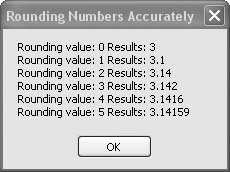# Recipe 6.8. Rounding Numbers Accurately

#### Problem

You need to round off double-precision numbers in a standard, accurate way.

#### Solution

Sample code folder: Chapter 06\Rounding

Use the Math.Round() function to round numbers to the desired precision.

#### Discussion

The Math.Round() function is overloaded to accept several different sets of parameters. If you pass just a Double or Decimal number to it, the number is rounded to the nearest whole number. By passing a second parameter, you control the number of digits after the decimal point where the rounding is to occur. For example, the following code rounds off the value of pi (π) using zero through five as the number of digits for the rounding:

` Dim outputFormat As String = _    "Rounding value: {0} Results: {1}" Dim oneTry As String Dim result As New System.Text.StringBuilder Dim piRounded As Double Dim digits As Integer For digits = 0 To 5    piRounded = Math.Round(Math.PI, digits)    oneTry = String.Format(outputFormat, digits, piRounded)    result.AppendLine(oneTry) Next digits MsgBox(result.ToString()) `

Figure 6-8 shows the results of these rounding actions.

##### Figure 6-8. Using the Math.Round( ) function to round numbers accuratelyA third optional parameter lets you fine-tune the way a number is rounded when the number is exactly halfway between two values at the point where the number is to be rounded. The choices are to have the number rounded to an even digit, or away from zero. The default is to round to an even digit.